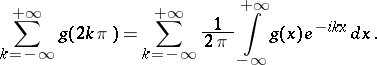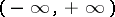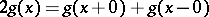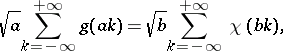# Poisson summation formula

(diff) ← Older revision | Latest revision (diff) | Newer revision → (diff)

The formulaThe Poisson summation formula holds if, for example, the functionis absolutely integrable on the interval, has bounded variation and. The Poisson summation formula can also be written in the formwhereandare any two positive numbers satisfying the condition, andis the Fourier transform of the function:How to Cite This Entry:
Poisson summation formula. Encyclopedia of Mathematics. URL: http://encyclopediaofmath.org/index.php?title=Poisson_summation_formula&oldid=13421
This article was adapted from an original article by I.I. Volkov (originator), which appeared in Encyclopedia of Mathematics - ISBN 1402006098. See original article